# Collisionality Parameters

Consider an equilibrium magnetic flux-surface whose label is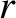. Let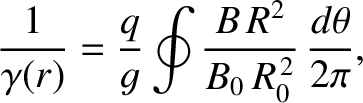(A.8)

where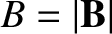, and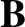is the equilibrium magnetic field. Here,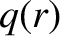,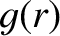,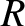,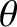,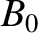, andare specified in Sections 14.2 and 14.4. It is helpful to define a new poloidal angle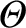such that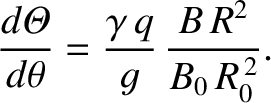(A.9)

Let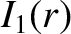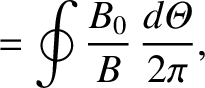(A.10)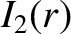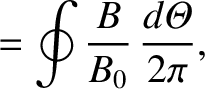(A.11)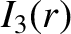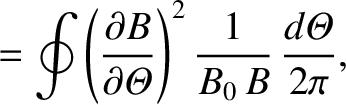(A.12)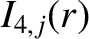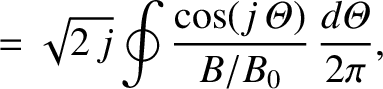(A.13)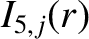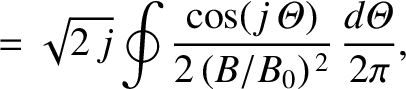(A.14)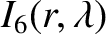(A.15)

where the integrals are taken at constant,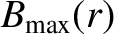is the maximum value of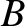on the magnetic flux-surface, and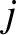a positive integer. The species-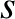transit frequency is written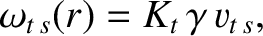(A.16)

where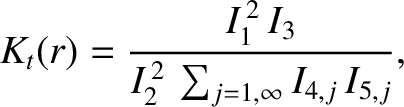(A.17)

and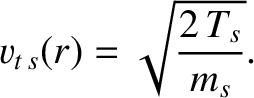(A.18)

Here,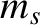is the species-mass, and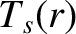the species-temperature (in energy units). The fraction of passing particles is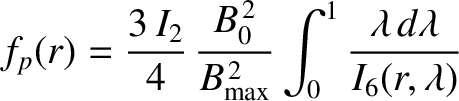(A.19)

[See Equation (2.200).] Finally, the dimensionless species-collisionality parameter [see Equation (2.95)]. is written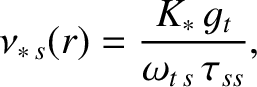(A.20)

where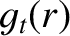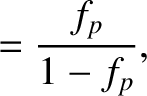(A.21)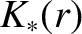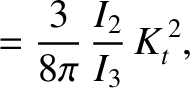(A.22)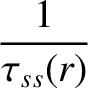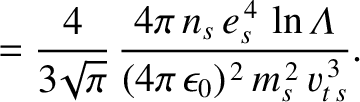(A.23)

[See Equation (2.190).] Here, the Coulomb logarithm,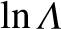, is assumed to take the same large constant value (i.e.,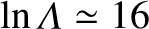), independent of species.